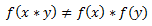# Answer to Question #4997 in Abstract Algebra for Annemarie

Question #4997
Consider the function f : Z4 &rarr; Z12 defined by f(x) = 3x. Show that f(x) does
NOT preserve multiplication.
1
2011-11-08T09:33:31-0500
f:Z4 --&gt; Z12, f(x)=3x
We will show that this function does not preserve multiplication. we can consider x,y from Z4 such thatlet x=1, y=3 so we have:
f(1*3)=f(3)=9 (modulo 12)
f(1)*f(3)=3*9=27=3( modulo 12)
But 9 is not equal to 3 in Z12. So f does not preserve multiplication.

Need a fast expert's response?

Submit order

and get a quick answer at the best price

for any assignment or question with DETAILED EXPLANATIONS!